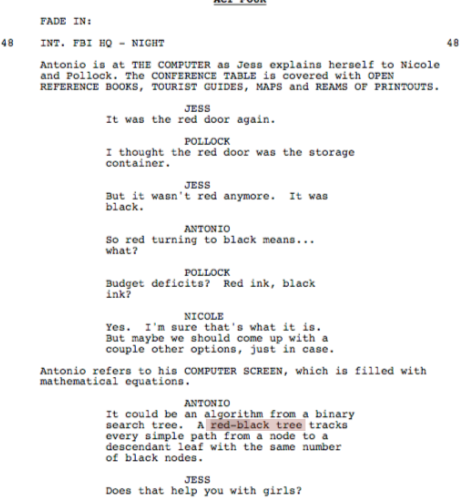# [Algorithms I] Week 5-1 Balanced Search Trees

goal: lgN for insert/search/delete operations (not necessarily binary trees..)
3 algo: 2-3 tree, (left leaning) red-black tree, B-tree

# 1. 2-3 Search Trees

def. 2-3 tree

• allow 1 or 2 keys per node, & 2 or 3 children per node:
• 2-node: one key, 2 children (ordinary BST node)
• 3-node: 2 keys, 3 children (3 children: less, between, more)
• perfect balance: every path from root to null link has the same length (2-3 tree的一个超好的性质, 类似于一个满二叉树!)
• symmetric order: inorder traversal gives ascending order (和BST类似)search

insert

• case 1: insert into a 2-node at bottom

just convert a 2-node into a 3-node

• case 2: insert into a 3-node at bottom
• create a temporary 4-node (three keys)
• move middle key in 4-node into parent, split the rest two keys into two 2-nodes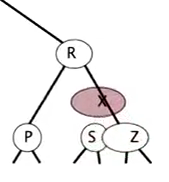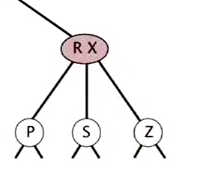• if parent becom a 3-node → continue the process
• if arrived at the root (root is a 4-node with three keys): split it into three 2-nodes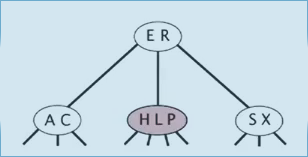splitting a 4-node: can be done in constant time (local transformation).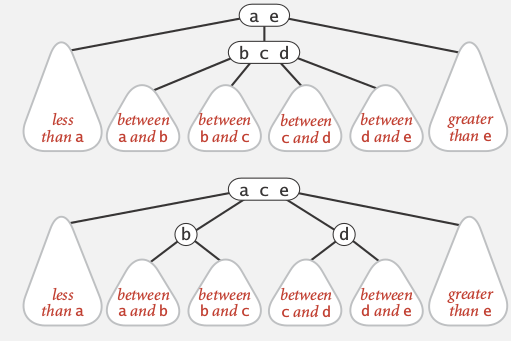## Analysis

Invariant: maintains symmetric order and perfect balance.
proof.
each transformation maintains the order and the balance, all possible transformations:performance
every path from root to null link has the same length.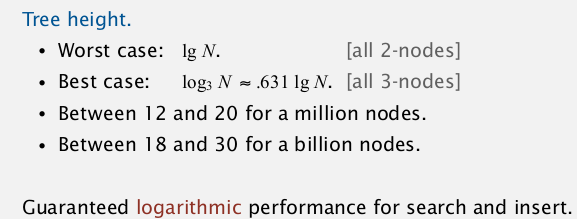## Implementation

• direct implementation is complicated:
• bottom line: Could do it, but there's a better way.

# 2. Red-Black BST

LLRB tree: left-leaning red-black tree.

BST representation of the 2-3 trees
use internal left-leaning links for 3 nodes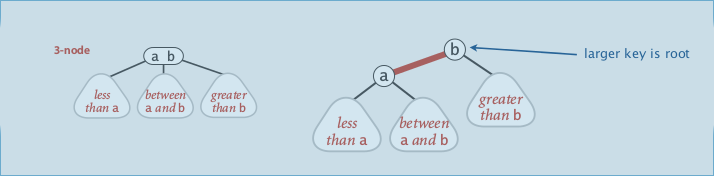or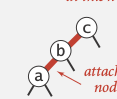orexample: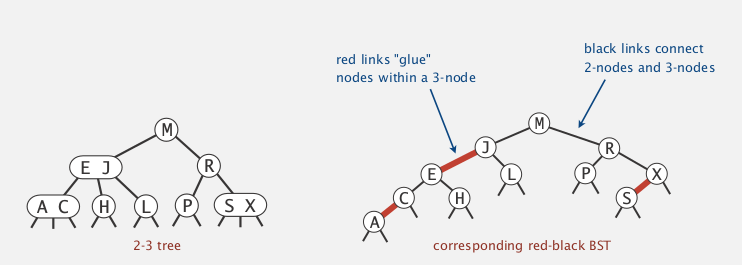## properties

• every path from path to null link has the same number of black links (想象所有red link都变为horizontal)
• all red links lean left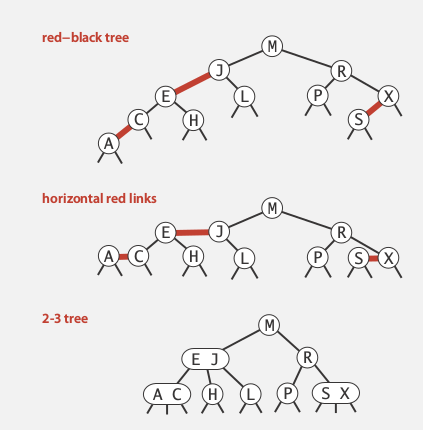## representation

Each node has only one link from parent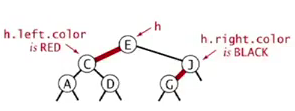```private class Node{
private Key key;
private Value val;
Node left, right;
boolean color;//true means red
}
private boolean isRed(Node nd){
if (nd==null) return false;
return nd.color;
}
```

insert to parent 操作: 只需把color变为RED即表示该节点 被变成了和父节点一起的一个(虚拟)节点.

## elementary operations

left-rotation
(def: convert a right-learning red link to left. )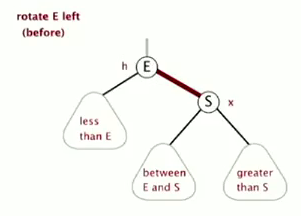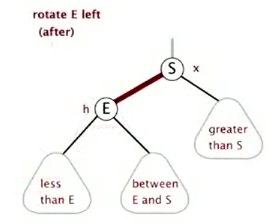(symmetric ordering and perfect black balance are maintained)

```private Node rotateLeft(Node h){
Node s = h.right;
h.right = s.left;
s.left = h;
s.color = h.color;   // not = BLACK
h.color = RED;
return s;
}
```

right-rotation
(temporarily turn a left-leaning red link to right)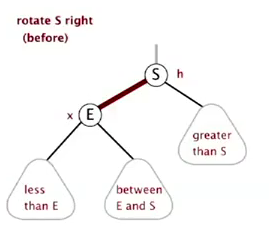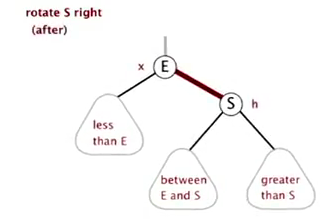`private Node rotateRight(Node h){...}`

right rotation 是为了应对这种情况: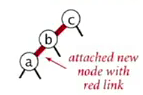rotateRight(c) ⇒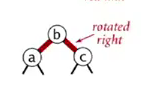color-flip
(split a 4-node, with three kyes — two red links)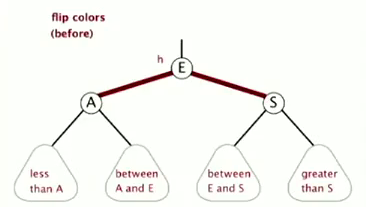```private void filpColor(Node h){
h.color = RED;
h.left.color = BLACK;
h.right.color = BLACK;
}
```

## Implementation

Basic strategy
Maintain one-to-one correspondence with 2-3 tree by applying elementary operations.

• search

Exactly the same as elementary BST. ( ⇒ The same code for floor and ceiling)

• insert

Each insert will generate a red link (then should rotate to make it legal)

1. insert into a 2-node at the bottom• standart BST insert
• if have red right link: rotateLeft

ex: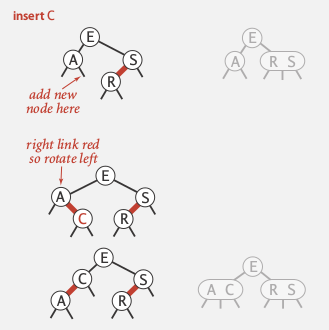1. insert into a 3-node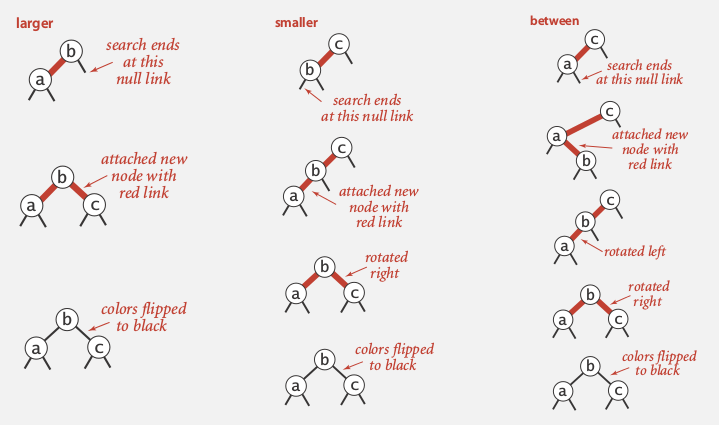• standard BST insert and color nodes
• if necessary, rotate to balance 4-node, 比如: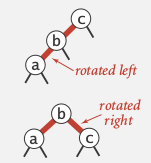• flip colors to pass red link to upper level
• if necessary, rotate to make all links left-leaning

ex: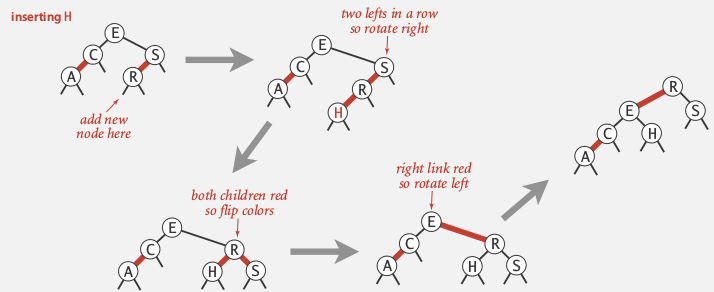ex2: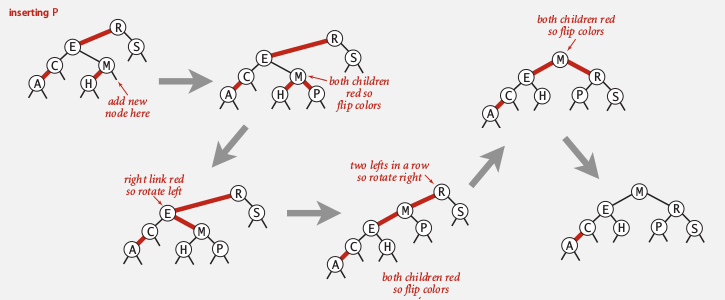## Code

1. left = black, right = red⇒ rotateLeft(a)

1. left =red, left.right = red [这个不会出现, 因为这对于下一层来说是case 1..]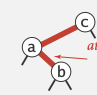⇒ rotateLeft(e) ⇒ 变为case 3

1. left = red, left.left = red⇒ rotateRight(s) ⇒ 变为case 4

1. left = red. right = red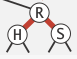⇒ flipColor(r)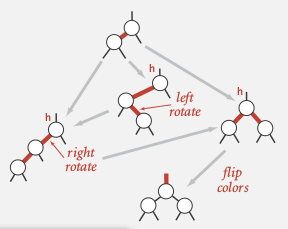(这个也是在2007年algo第四版的时候才刚刚弄出来的, 以前的代码要复杂)

```private Node put(Node nd, Key k, Value v){
if(nd==null) return new Node(k,v,RED);
int cmp = k.compareTo(nd.key);
if(cmp==0) nd.val = v; // 这里不急着返回 -- same trick as for BSTs..
else if(cmp<0) nd.left = put(nd.left, k, v);
else nd.right = put(nd.right, k, v);
// modifications to maintain LLRB tree property:
if( isRed(nd.right) && !isRed(nd.left) ) nd = rotateLeft(nd);//case 1
//if( isRed(nd.left) && isRed(nd.left.right) ) nd.left = rotateLeft(nd.left);// case 2 -- never happen...
if( isRed(nd.left) && isRed(nd.left.right) ) nd = rotateRight(nd);// case 3
if( isRed(nd.left) && isRed(nd.right) ) flipColor(nd);//case 4
return nd;
}
```

## Analysis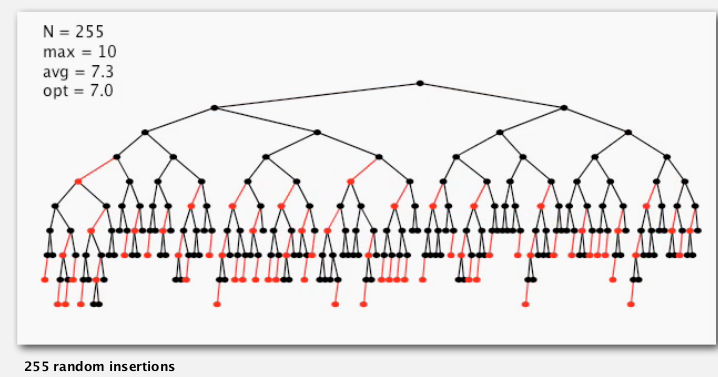worst case: the left path is alternating red and black.
⇒ longest path <= 2 * shortest path (height<= 2lgN)

practical applications: height ~ 1.0 lgN

summery:# 3. B-trees

setting: data access in file system.
Probe is much expensive than accessing data within a page.

Goal: access data using a minimum number of probes.

## B-tree

def.
external nodes: contain just keys, not links

def. B-tree
Generalize 2-3 trees by allowing up to M-1 keys per node:

• = 2 keys in root

• = M/2 keys in other nodes

• external nodes contain client keys
• internal nodes contain copies of keys to guide search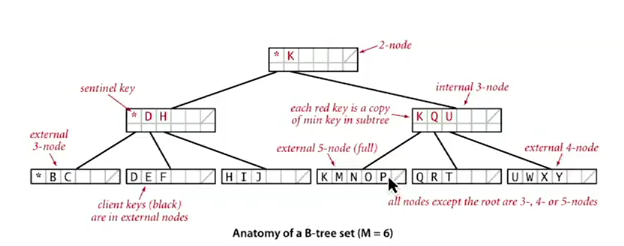## Searching

similar to BST/2-3tree
ex.(Choose M as large as possible so that M links fit into a page)

## Insertion

similar to 2-3 tree## Analysis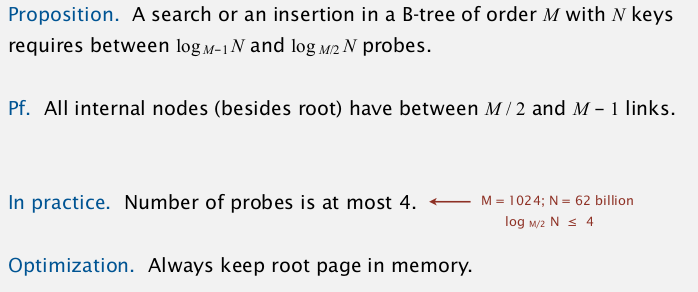## System implementations

system implementations of RBtree.
java:
`java.util.TreeMap`, `java.util.TreeSet`.